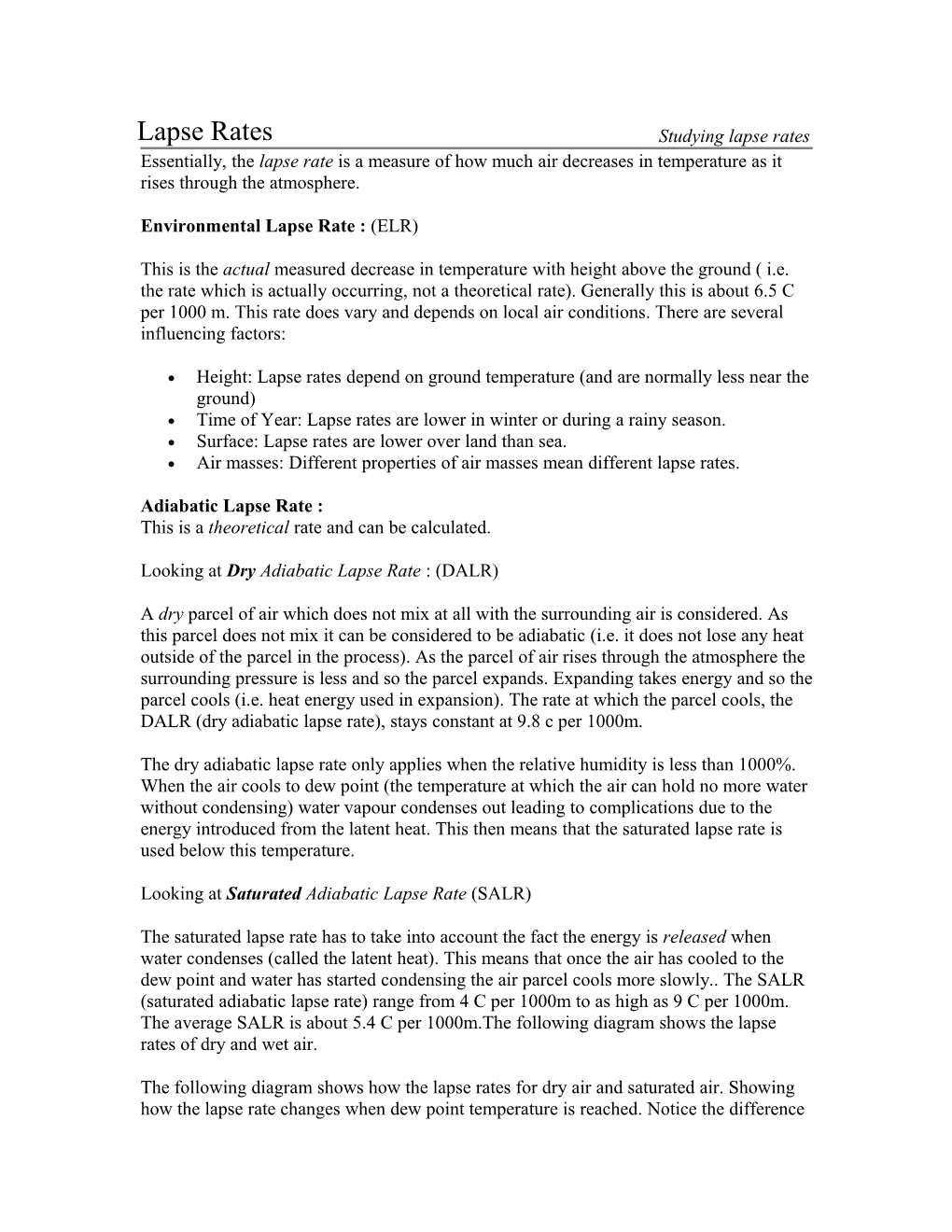# Environmental Lapse Rate : (ELR)Lapse Rates / Studying lapse rates

Essentially, the lapse rate is a measure of how much air decreases in temperature as it rises through the atmosphere.

Environmental Lapse Rate : (ELR)

This is the actual measured decrease in temperature with height above the ground ( i.e. the rate which is actually occurring, not a theoretical rate). Generally this is about 6.5 C per 1000 m. This rate does vary and depends on local air conditions. There are several influencing factors:

• Height: Lapse rates depend on ground temperature (and are normally less near the ground)
• Time of Year: Lapse rates are lower in winter or during a rainy season.
• Surface: Lapse rates are lower over land than sea.
• Air masses: Different properties of air masses mean different lapse rates.

Adiabatic Lapse Rate :
This is a theoretical rate and can be calculated.

Looking at Dry Adiabatic Lapse Rate : (DALR)

A dry parcel of air which does not mix at all with the surrounding air is considered. As this parcel does not mix it can be considered to be adiabatic (i.e. it does not lose any heat outside of the parcel in the process). As the parcel of air rises through the atmosphere the surrounding pressure is less and so the parcel expands. Expanding takes energy and so the parcel cools (i.e. heat energy used in expansion). The rate at which the parcel cools, the DALR (dry adiabatic lapse rate), stays constant at 9.8 c per 1000m.

The dry adiabatic lapse rate only applies when the relative humidity is less than 1000%. When the air cools to dew point (the temperature at which the air can hold no more water without condensing) water vapour condenses out leading to complications due to the energy introduced from the latent heat. This then means that the saturated lapse rate is used below this temperature.

Looking at Saturated Adiabatic Lapse Rate (SALR)

The saturated lapse rate has to take into account the fact the energy is released when water condenses (called the latent heat). This means that once the air has cooled to the dew point and water has started condensing the air parcel cools more slowly.. The SALR (saturated adiabatic lapse rate) range from 4 C per 1000m to as high as 9 C per 1000m. The average SALR is about 5.4 C per 1000m.The following diagram shows the lapse rates of dry and wet air.

The following diagram shows how the lapse rates for dry air and saturated air. Showing how the lapse rate changes when dew point temperature is reached. Notice the difference in steepness between the DALR and the SALR. The SALR means that the air cools less for the same rise in height.

The differecnes between lapse rates in the atmosphere enable different weather to occur and different clouds form. See stability and instability

Summary:

ELR / Environmental Lapse Rate / Actual lapse rate measured in the air
DALR / Dry Adiabatic Lapse Rate / Theoretical lapse rate for dry air (ie air below dew point)
SALR / Saturated Adiabatic Lapse Rate / Theoretical lapse rate for saturated air, ie with vapour condensing